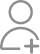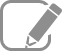Take Class 9 Tuition from the Best Tutors

•Affordable fees
•1-1 or Group class
•Flexible Timings
•Verified Tutors

Search in

The volume of a right circular cone is 9856 cm3 . If the diameter of the base is 28 cm,find(i) height of the cone(ii) slant height of the cone(iii) curved surface area of the cone

Learn Exercise 13.7Follow 4Answer(i) Radius of cone = Let the height of the cone be h. Volume of cone = 9856 cm3 h = 48 cm Therefore, the height of the cone is 48 cm. (ii) Slant height (l) of cone Therefore, the slant height of the cone is 50 cm. (iii) CSA of cone = πrl = 2200 cm2 Therefore, the curved surface area of the cone...

(i) Radius of cone =Let the height of the cone be h.

Volume of cone = 9856 cm3h = 48 cm

Therefore, the height of the cone is 48 cm.

(ii) Slant height (l) of coneTherefore, the slant height of the cone is 50 cm.

(iii) CSA of cone = πrl= 2200 cm2

Therefore, the curved surface area of the cone is 2200 cm2.

48cm. 50cm. 2200cm sq

Professional in Adaptive Learning for 8-12th with 11 Yrs of Experience in Quality Teaching

1) height = 48 cm 2) slant height= 50 cm 3) curved surface area = 2200 sq cm

Professional in Adaptive Learning for 8-12th with 11 Yrs of Experience in Quality Teaching

1) height = 48 cm 2) slant height= 50 cm 3) curved surface area = 2283.69sq m

Experienced Maths Teacher

(i)V = 1/3 * π* r*r*h 9856 = 1/3 *(22/7) *14*14*h h= (9856*3*7)/(22*14*14) =48 cm (ii) slant height l = √(r*r + h*h) = √ (196+2304) = √2500 = 50 cm (iii) curved surface area π*r*l = (22/7)*14*50 =2200 cm^2

(i)V = 1/3 * π* r*r*h

9856 = 1/3 *(22/7) *14*14*h

h=  (9856*3*7)/(22*14*14)

=48 cm

(ii) slant height

l = √(r*r + h*h) = √ (196+2304)

= √2500 = 50 cm

(iii) curved surface area

π*r*l = (22/7)*14*50

=2200 cm^2

Related Questions13/09/2019
618/08/2019
5

Now ask question in any of the 1000+ Categories, and get Answers from Tutors and Trainers on UrbanPro.com

Find Class 9 Tuition near you

Looking for Class 9 Tuition ?

Learn from the Best Tutors on UrbanPro

Are you a Tutor or Training Institute?

Join UrbanPro Today to find students near you
X

Looking for Class 9 Tuition Classes?

The best tutors for Class 9 Tuition Classes are on UrbanPro

• Select the best Tutor
• Book & Attend a Free Demo
• Pay and start LearningTake Class 9 Tuition with the Best Tutors

The best Tutors for Class 9 Tuition Classes are on UrbanProUrbanPro.com is India's largest network of most trusted tutors and institutes. Over 55 lakh students rely on UrbanPro.com, to fulfill their learning requirements across 1,000+ categories. Using UrbanPro.com, parents, and students can compare multiple Tutors and Institutes and choose the one that best suits their requirements. More than 7.5 lakh verified Tutors and Institutes are helping millions of students every day and growing their tutoring business on UrbanPro.com. Whether you are looking for a tutor to learn mathematics, a German language trainer to brush up your German language skills or an institute to upgrade your IT skills, we have got the best selection of Tutors and Training Institutes for you. Read more